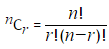### The Problem

There are exactly ten ways of selecting three from five, 12345:

123, 124, 125, 134, 135, 145, 234, 235, 245, and 345

In combinatorics, we use the notation, 5C3 = 10.

In general,,where r ≤ n, n! = n×(n−1)×...×3×2×1, and 0! = 1.

It is not until n = 23, that a value exceeds one-million: 23C10 = 1144066.

How many, not necessarily distinct, values of nCr, for 1 ≤ n ≤ 100, are greater than one-million?

### The Solution

Thankfully, the problem definition has already given us the hard part of this problem. That is, figuring out the number of combinations for nCr.

The words "in general" in the problem definition made me nervous, but I couldn't think of any special cases that would apply here.

``````let rec fact n =
match n with
| 0 -> 1I
| 1 -> 1I
| _ -> bigint(n) * fact (n-1)

let rec numCombos n r =
fact n / ((fact r) * (fact (n - r)))
``````

The `numCombos` function returns the number of combinations for a given `n` and `r`.

Now we can solve the problem:

``````seq {
for n in 1..100 do
for r in 1..n do
yield (n,r)
}
|> Seq.map (fun (n,r) -> numCombos n r)
|> Seq.filter (fun n -> n > 1000000I)
|> Seq.length
``````

The problem definition specifies 1 ≤ n ≤ 100. Therefore 1 ≤ r ≤ n because you can't take more from n than n.

We'll start by generating a sequence of all possible `n` paired with the possible `r` values for that `n`.

Next, we generate the number of combinations for each `(n,r)` pair.

Finally we filter out the values where the number of combos is more than one million and count them. That is the answer.

Other Posts in This Series